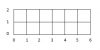BrainDen.com - Brain Teasers
• 0# Monochromatic rectangle on a two-colored plane

## Question

This problem is adapted from a problem given in the 1976 USA Mathematics Olympiad.

Prove or disprove:

Any 2-colored plane contains an m x n monochromatic rectangle (4 vertices of same color) such that

1. m = 1 or 2, and
2. n <= 6.

## Recommended Posts

• 0Consider coloring the corners and intersections in this figure, either red or blue. Suppose it can be done so that no monochromatic rectangle exists.

Let (a,b) denote column a, row b, according to the numbers in the figure. If any column has all three points of the same color, change one of them to the other color. For example, if (3,0), (3,1), and (3,2) are all red, change one of them into blue. This change can never result in a new monochromatic rectangle appearing, so we still have no monochromatic rectangles. Repeat this process until all columns have both colors. There are six possible coloring patterns for a column: RRB, RBR, RBB, BBR, BRB, and BRR. However, there are seven columns, so two of them must have the same pattern. This will yield a monochromatic rectangle. For example, if columns 2 and 5 are both RRB, then taking the two red points from each column yields the rectangle (2,0),(2,1),(5,1),(5,0) which is all red and is of size 1x3. So there will always be a monochromatic rectangle in this figure. Finally note that any monochromatic rectangle from this figure is of requested size.

## Join the conversation

You can post now and register later. If you have an account, sign in now to post with your account.×   Pasted as rich text.   Paste as plain text instead

Only 75 emoji are allowed.

×   Your previous content has been restored.   Clear editor

×   You cannot paste images directly. Upload or insert images from URL.

×